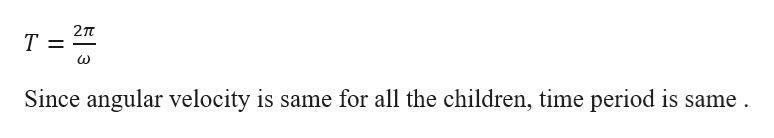5)Four children are sitting on a merry-go-round at different distances from the center (axis of rotation) indicated as A, B, C, and D locations with A being the closest to the center.  Choose all the correct statements from the list below. A.For every rotation, the distance traveled by a child is 2πR, where R is the distance from the center. B.All children have a centripetal acceleration pointing towards the center. C.Child A is moving the fastest. D.All children have the same period, T. E.All children have a different angular velocity based on their distance from the center. F.All children have the same angular speed regardless of their distance from the center. G.Child D is moving the fastest tangentially since he/she is the farthest from the center.

Question

5)

Four children are sitting on a merry-go-round at different distances from the center (axis of rotation) indicated as A, B, C, and D locations with A being the closest to the center.  Choose all the correct statements from the list below.

 A. For every rotation, the distance traveled by a child is 2πR, where R is the distance from the center. B. All children have a centripetal acceleration pointing towards the center. C. Child A is moving the fastest. D. All children have the same period, T. E. All children have a different angular velocity based on their distance from the center. F. All children have the same angular speed regardless of their distance from the center. G. Child D is moving the fastest tangentially since he/she is the farthest from the center.
Step 1

A) correct
Since all the points are moving circular path , distance travelled is 2(pi)R.
B) correct
In order to make an object move in a circular path we apply centripetal force and the bodies will experience centripetal acceleration.
Direction of centripetal acceleration is towards centre of rotation.
C) Wrong Statement
Child A is close to the centre , since radius of rotation is less , velocity of child A will be less.
Velocity (v) = (radius)*(Angular Velocity)

Step 2

D) Correct
All the children will ...help_outlineImage Transcriptionclose2TT T 1 Since angular velocity is same for all the children, time period is same fullscreen

Want to see the full answer?

See Solution

Want to see this answer and more?

Our solutions are written by experts, many with advanced degrees, and available 24/7

See Solution
Tagged in

Angular Motion